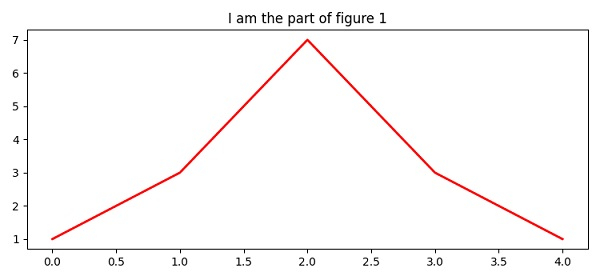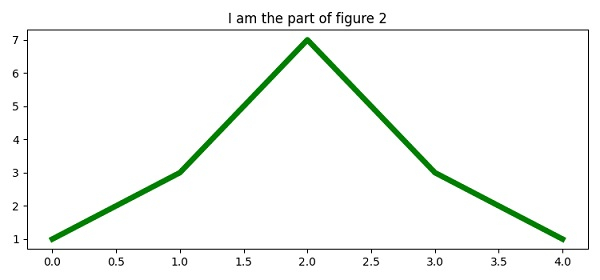# How can I show figures separately in Matplotlib?

To show multiple figures in matplotlib, we can take the following Steps −

• To create a new figure, or activate an existing figure, use the figure() method. (Create two figures namely, Figure1 and Figure2).

• Plot the lines with the same lists (colors red and green and linewidth 2 and 5).

• Set the title of the plot over both the figures.

• To display the figure, use the show() method.

## Example

from matplotlib import pyplot as plt
plt.rcParams["figure.figsize"] = [7.50, 3.50]
plt.rcParams["figure.autolayout"] = True
fig1 = plt.figure("Figure 1")
plt.plot([1, 3, 7, 3, 1], c="red", lw=2)
plt.title("I am the part of figure 1")
fig2 = plt.figure("Figure 2")
plt.plot([1, 3, 7, 3, 1], c="green", lw=5)
plt.title("I am the part of figure 2")
plt.show()

## Output